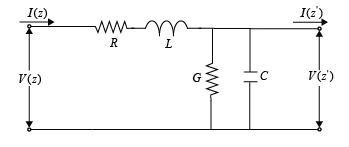# txlineRLCGLine

Create RLCG line transmission line

Since R2020b

## Description

Use the `txlineRLCGLine` object to create a RLCG transmission line that is characterized by line loss, line length, stub type, and termination.## Creation

### Syntax

``rlcgtxline = txlineRLCGLine``
``rlcgtxline = txlineRLCGLine(Name=Value)``

### Description

````rlcgtxline = txlineRLCGLine` creates a default RLCG line transmission line object.```

example

````rlcgtxline = txlineRLCGLine(Name=Value)` sets Properties using one or more name-value pairs. For example, ```rlcgtxline = txlineRLCGLine(Freq=2.0e9)``` creates an RLCG line transmission line at a frequency of 2 GHz.. Properties you do not specify retain their default values.```

## Properties

expand all

Name of the RLCG line transmission line, specified as a string scalar or a character vector.

Example: `Name='rlcgline1'`

Example: `rlcgtxline.Name = 'rlcgline1'`

Data Types: `char` | `string`

Frequency of the RLCG of the transmission line, specified as a nonnegative scalar in hertz.

Example: `Freq=2.0e9`

Example: `rlcgtxline.Freq = 2.0e9`

Data Types: `double`

Resistor values per length of the transmission line, specified as a nonnegative scalar in ohms per meter or a nonnegative vector with each element unit in ohms per meter.

Example: `R=0.5`

Example: `rlcgtxline.R = 0.5`

Data Types: `double`

Inductance values per length of the transmission line, specified as a nonnegative scalar in Henry per meter or a nonnegative vector with each element unit in Henry per meter.

Example: `L=0.5`

Example: `rlcgtxline.L = 0.5`

Data Types: `double`

Capacitance values per length of the transmission line, specified as a nonnegative scalar in Faraday per meter or a nonnegative vector with each element unit in Faraday per meter.

Example: `C=0.5`

Example: `rlcgtxline.C = 0.5`

Data Types: `double`

Conductance values per length of the transmission line, specified as a nonnegative scalar in Siemens per meter or a nonnegative vector with each element unit in Siemens per meter.

Example: `G=0.5`

Example: `rlcgtxline.G = 0.5`

Data Types: `double`

Type of interpolation, specified as `'Linear'`, `'Spline'`, or `'Cubic'`.

Example: `IntpType=Spline'`

Example: `rlcgtxline.IntpType = 'Spline'`

Data Types: `char`

Physical length of the transmission line, specified as a positive scalar in meters.

Example: `LineLength=,0.0200`

Example: `rlcgtxline.LineLength = 0.0200`

Data Types: `double`

Stub transmission line termination, specified as `'NotApplicable'`, `'Open'`, or `'Short'`.

Example: `Termination='Short'`

Example: `rlcgtxline.Termination = 'Short'`

Data Types: `char`

Type of stub, specified as `'NotAStub'`, `'Series'`, or `'Shunt'`.

Example: `StubMode='Series'`

Example: `rlcgtxline.StubMode = 'Series'`

Data Types: `char`

Number of input and output ports, specified as positive scalar.

Data Types: `double`

Number of input and output ports, specified as a cell array of strings.

Data Types: `char` | `string`

## Object Functions

 `sparameters` Calculate S-parameters for RF data, network, circuit, and matching network objects `groupdelay` Group delay of S-parameter object or RF filter object or RF Toolbox circuit object `noisefigure` Calculate noise figure of transmission lines, series RLC, and shunt RLC circuits `getZ0` Calculate characteristic impedance with and without dispersion for transmission line `circuit` Circuit object `clone` Create copy of existing circuit element or circuit object

## Examples

collapse all

Create an RLCG transmission line using these specifications:

• Resistor : 100 ohms

• Capacitor : 1 pF

`rlcglinetxline = txlineRLCGLine(R=100,C=1e-12)`
```rlcglinetxline = txlineRLCGLine: RLCGLine element Name: 'RLCGLine' Frequency: 1.0000e+09 R: 100 L: 0 C: 1.0000e-12 G: 0 IntpType: 'Linear' LineLength: 0.0100 Termination: 'NotApplicable' StubMode: 'NotAStub' NumPorts: 2 Terminals: {'p1+' 'p2+' 'p1-' 'p2-'} ```

Calculate the S-parameters of the transmission line at 1 GHz.

`sparam = sparameters(rlcglinetxline,1e9);`

## Version History

Introduced in R2020b